This is the graphical debugger. It visualizes a series of RSX commands that had been dumped using the `dbg-gui`.

A frame can be reproduced command-by-command and the complete RSX state and all the associated textures and shaders can be viewed after each command.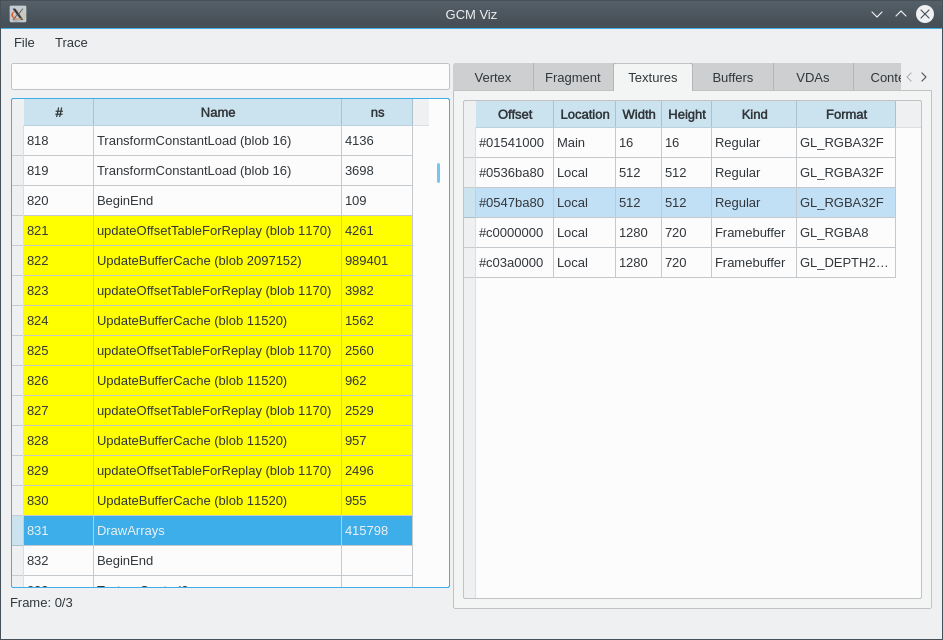For example the following frame was produced using several hundred `DrawArrays` commands.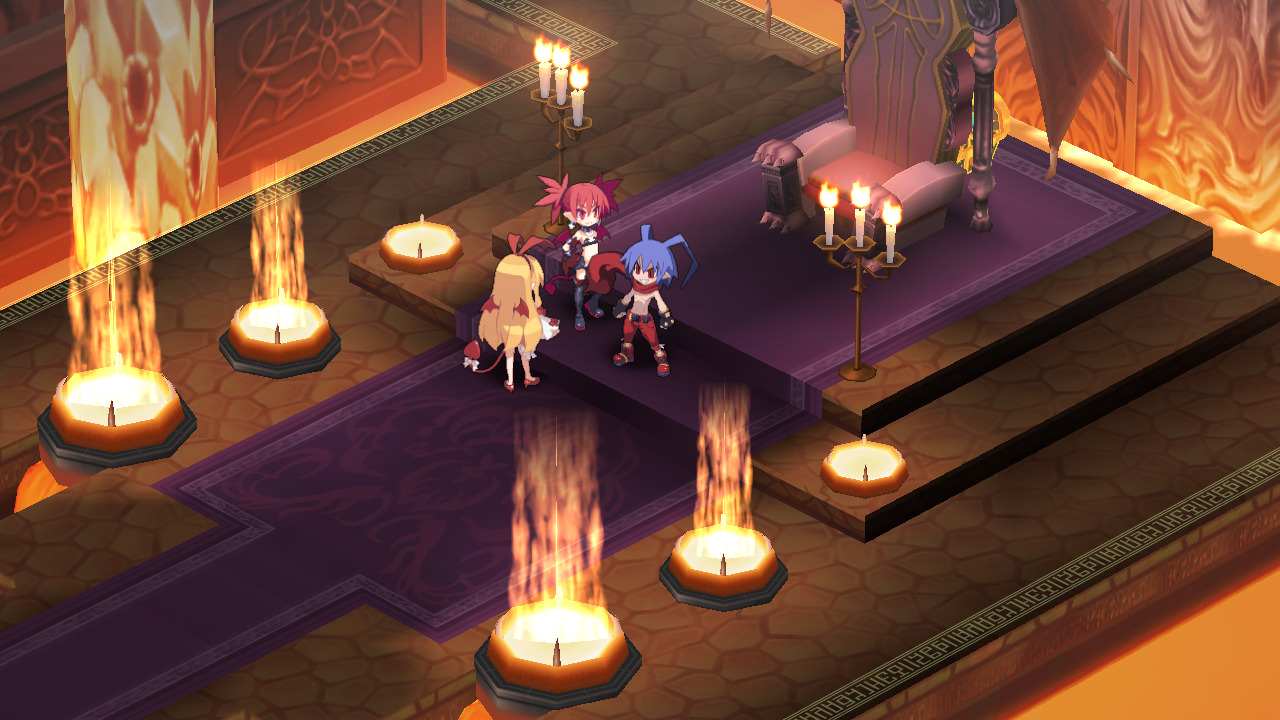After the first several draw commands the following part of the frame was drawn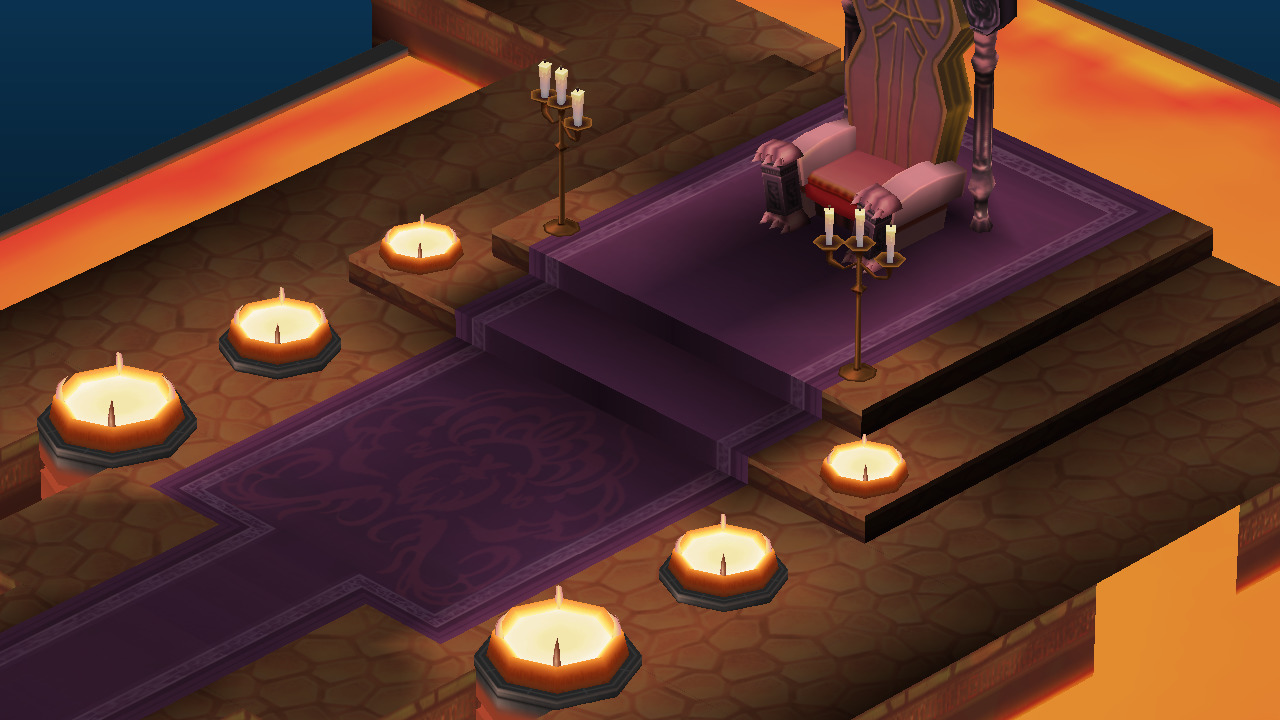To do so two textures were used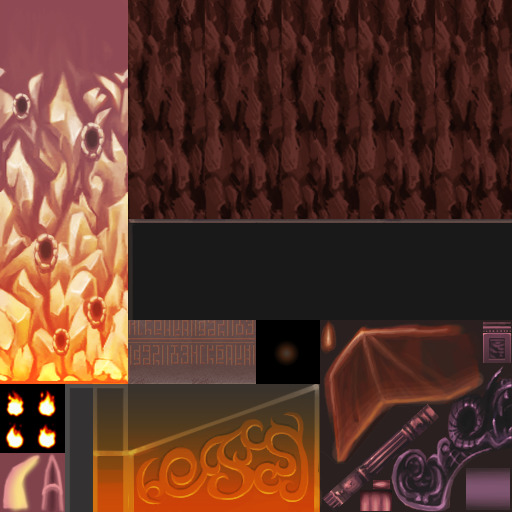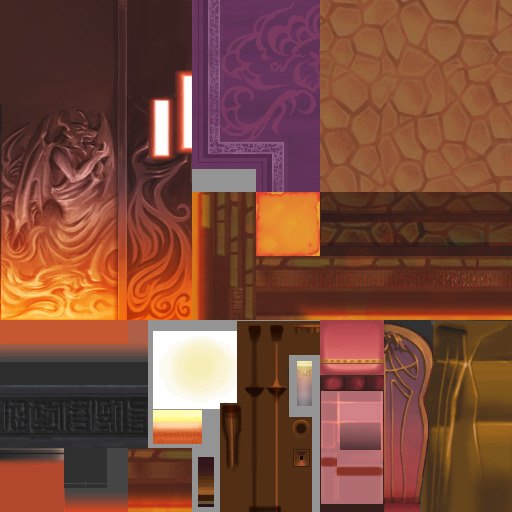``````000|000: TEXR R1, f[TEX0], TEX0;
001|001: MOVH H0, f[COL0];
002|002: FENCTR;
003|003: MULR R2, H0, R1;
004|004: FENCTR;
005|005: MULR R2, R2, f[TEX2];
006|006: MOVR R0.w, R2;
007|007: MULR R0.xyz, f[TEX1], H0;
008|008: MOVR R1.xyz, R1;
009|009: MADR R0.xyz, R0, R1, R2; # last instruction
``````

That in turn was translated into the following (abridged) GLSL

``````r = tex0(f_TEX0);
h = f_COL0;
while (false) { }
r = (h * r);
while (false) { }
r = (r * f_TEX2);
r.w = r.w;
r.xyz = (f_TEX1 * h).xyz;
r.xyz = r.xyz;
r.xyz = ((r * r) + r).xyz;
``````

It is also possible to see e.g. how the Surface was configured, what vertex data attributes were used and what their formats are.